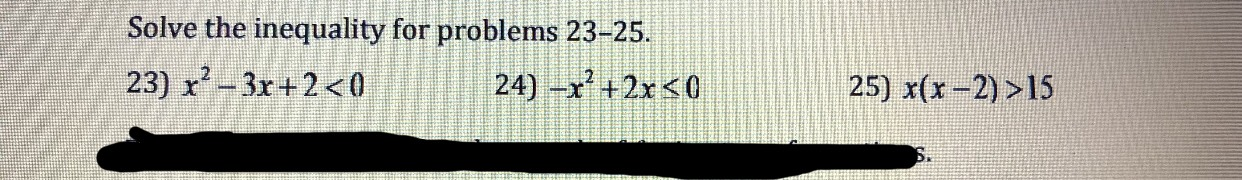# Solve the inequality for problems 23-25.25) x(x-2) >15

Question
6 views

For 25help_outlineImage TranscriptioncloseSolve the inequality for problems 23-25. 25) x(x-2) >15 fullscreen
check_circle

Step 1

We have been given an inequality x(x-2)>15 and we need to solve it. In order to solve this inequality, we will bring all the terms on the same side and then we will simplify it before factoring again. Once we have the factored, we can easily test the intervals for the final answer.

Here is how the inequality would look like after carrying all the terms on the same side and factoring.

Step 2

Our critical numbers from the inequality are x = -3 and x = 5, which split the entire numberline into 3 intervals, namely, (-inf, -3), (-3, 5) and (5, inf).

Now we can choose a value from each interva...

### Want to see the full answer?

See Solution

#### Want to see this answer and more?

Solutions are written by subject experts who are available 24/7. Questions are typically answered within 1 hour.*

See Solution
*Response times may vary by subject and question.
Tagged in

### Equations and In-equations